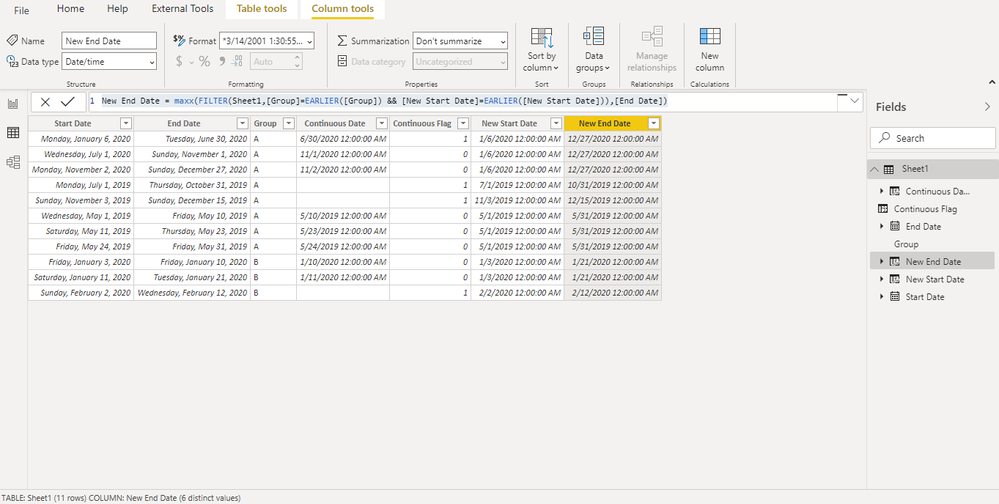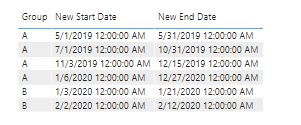cancel
Showing results for
Did you mean:

Fabric is Generally Available. Browse Fabric Presentations. Work towards your Fabric certification with the Cloud Skills Challenge.## Power BI Continuous Streak - With One-Day Break

Problem Description:

There is a set within a group with start and end dates. When the end date and next start date have a gap of only one day, these dates need to be combined.

Note: In HR, these one-day gap sets can be employee leave start and end date.

Solution: For the solution, we need to create the partition of the continuous dates.

Steps:

1. Create a flag to check that the dates are continuous. This flag needs to be bi-directional for a statement/line to check only 1-day gap on either side. We will get two columns:

``````Continuous Flag = var _1 = DATEDIFF(Sheet1[End Date]+1 , MINX(FILTER(Sheet1, ([Start Date]=EARLIER(Sheet1[End Date])+1 )&& [Group]=EARLIER(Sheet1[Group]) ),[Start Date]),DAY) +DATEDIFF( Sheet1[Start Date] -1, maxx(FILTER(Sheet1, ( [end Date]=EARLIER(Sheet1[Start Date])-1)  && [Group]=EARLIER(Sheet1[Group])) ,[End Date]),DAY)
// var _2=[Date Rank] -  maxx(FILTER(Sheet1, ( [Date Rank]=EARLIER([Date Rank])-1) ) && [Group]=EARLIER(Sheet1[Group])), [Date Rank])
Var _3 = datediff(maxx(FILTER(Sheet1,[End Date]<EARLIER([Start Date])  && [Group] =EARLIER(Sheet1[Group])),[End Date]),[Start Date].[Date],DAY)
return  _1 +if(_3<=1, BLANK(),1)+0``````

Date

``````Continuous Date = MAX( MINX(FILTER(Sheet1, ([Start Date]=EARLIER(Sheet1[End Date])+1 ) && [Group]=EARLIER(Sheet1[Group]) ),[Start Date]-1)
,maxx(FILTER(Sheet1, ( [end Date]=EARLIER(Sheet1[Start Date])-1)&& [Group]=EARLIER(Sheet1[Group])) ,[End Date]+1))``````

2. Using the above measures to calculate Start Date:

``````New Start Date = Var _1 = maxx(FILTER(Sheet1,[Group] =EARLIER(Sheet1[Group])  && [Continuous Date] <=EARLIER(Sheet1[Continuous Date])&& not(ISBLANK([Continuous Date])) && [Continuous Flag]<>EARLIER([Continuous Flag])),Sheet1[Start Date])
Var _2 = minx(FILTER(Sheet1,[Group] =EARLIER(Sheet1[Group]) && [Continuous Date] <=EARLIER(Sheet1[Continuous Date]) && [Continuous Flag]=EARLIER([Continuous Flag]) && not(ISBLANK([Continuous Flag])) ),Sheet1[Start Date])
Var _3 =SWITCH(True(),
[Continuous Flag]=1,[Start Date],
(ISBLANK(_1) && [Continuous Flag]=0),_2,
not(ISBLANK(_1)),_1,
[Start Date])
return if(ISBLANK(_3),[Start Date],_3)``````

3. Calculate an End Date with help from Start Date:

``New End Date = maxx(FILTER(Sheet1,[Group]=EARLIER([Group]) && [New Start Date]=EARLIER([New Start Date])),[End Date])``This is how final merged rows, look like:Let us know what you think about these measures. Share your thoughts on different use cases of the continuous streak.

You can get all my posts at https://community.powerbi.com/t5/Data-Stories-Gallery/Blog-Analysis/m-p/1265567#M4403